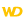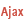Home |Sign in | EnglishHelpWLanguageWLanguage functionsControls, pages and windowsDrawing functionsPrefixed syntaxDrawing functions on WDPic variablesDrawPolygonPresentationExampleCoordinatesDrawing with opacity or anti-aliasingDrawing in PHPDrawing in Browser codeRelated examplesSee alsoOpenSaveAlphaBlendBackgroundChangeModeDrawArcDrawChordDrawCircleDrawLineDrawPointDrawPolygonDrawPolylineDrawRectangleDrawRectangleGradientDrawRoundedRectangleDrawSliceDrawTextDrawTextRTFEndDrawingFillFontHorizontalSymmetryInvertColorMergeLayerPixelColorPixelOpacityResizeRotationSaveStartDrawingVerticalSymmetryPicMergeLayerPicOpenPicResizePicRotationPicSaveNew WINDEV, WEBDEV 25 feature!
• Coordinates
• Drawing with opacity or anti-aliasing
• Drawing in PHP
• Drawing in Browser code
WINDEVWEBDEVWINDEV MobileOthers<WDPic variable>.DrawPolygon (Function)
In french: <Variable WDPic>.DessinePolygone
Draws a polygon in an WDPic variable.Versions 15 and laterThis function is now available for WEBDEV sites in Linux.New in version 15This function is now available for WEBDEV sites in Linux.This function is now available for WEBDEV sites in Linux.
Example
MyWDPicImage is WDPic = "Test.gif"
// Draw a 4-side polygon whose
// background is light yellow and whose border is light blue
MyWDPicImage.DrawPolygon(4, 10, 20, 20, 50, 40, 60, 50, 10, LightYellow, LightBlue)
IMG_MyDrawing = MyWDPicImage
Syntax

Building a polygon point by point

<WDPic image>.DrawPolygon(<Number of sides> , <X1> , <Y1> , <X2> , <Y2> [, <X3> , <Y3> [... [, <Xn> , <Yn>]]] [, <Background color> [, <Side color>]])
<WDPic image>: WDPic variable
Name of the WDPic variable to use. Only the background layer will be handled.
<Number of sides>: Integer
Number of sides in the polygon (up to 23 sides). This parameter defines the number of <X>, <Y> pairs to specify in the function.
<X1>: Integer
X coordinate of first point of the polygon. These coordinates are expressed in pixels.
<Y1>: Integer
Y coordinate of first point of the polygon. These coordinates are expressed in pixels.
<X2>: Integer
X coordinate of second point of the polygon. These coordinates are expressed in pixels.
<Y2>: Integer
Y coordinate of second point of the polygon. These coordinates are expressed in pixels.
<X3>: Integer
X coordinate of 3rd point of the polygon. These coordinates are expressed in pixels.
<Y3>: Integer
Y coordinate of 3rd point of the polygon. These coordinates are expressed in pixels.
<Xn>: Optional integer
X coordinate of nth point of the polygon. These coordinates are expressed in pixels.
<Yn>: Optional integer
Y coordinate of nth point of the polygon. These coordinates are expressed in pixels.
<Background color>: Integer or constant (optional)
Background color of polygon. This color can correspond to:
If this parameter is not specified, the background color:
<Side color>: Integer or constant (optional)
Color of polygon sides. This color can correspond to:
If this parameter is not specified, the line color:

Building a polygon via an array of X and Y coordinates

<WDPic image>.DrawPolygon(<Array of coordinates> [, <Background color> [, <Side color>]])
<WDPic image>: WDPic variable
Name of the WDPic variable to use. Only the background layer will be handled.
<Array of coordinates>: Array of integers or array of points
• WLanguage array of integers containing the coordinates of polygon points. The even subscript represent the X coordinates while the odd subscripts represent the Y coordinates of points. These coordinates are expressed in pixels.
•WLanguage array of Point variables containing the coordinates of polygon points. These coordinates are expressed in pixels.
<Background color>: Integer or constant (optional)
Background color of polygon. This color can correspond to:
• an RGB color (returned by RGB),
• an HSL color (returned by HSL),
• a preset color of WLanguage,
•a Color variable.
If this parameter is not specified, the background color:
<Side color>: Integer or constant (optional)
Color of polygon sides. This color can correspond to:
• an RGB color (returned by RGB),
• an HSL color (returned by HSL),
• a preset color of WLanguage,
•a Color variable.
If this parameter is not specified, the line color:
Remarks

Coordinates

Drawing a polygon:
MyImage is WDPic
MyImage.DrawPolygon(5, 80, 10, 10, 150, 100, 200, 160, 80, 140, 40)Coordinates are given with respect to the top left corner of the image (coordinates: (0.0)).
Important: There is no need to close the polygon (the coordinates of last point do not have to be equal to the coordinates of first point). The polygon is automatically "closed".
Limits: a polygon can contain up to 100 000 points in WINDEV, WEBDEV and Java, and up to 1000 points in Windows CE.Drawing with opacity or anti-aliasing

In the case of a drawing with opacity (<WDPic variable>.StartDrawing with the dWithOpacity constant) or anti-aliasing (<WDPic variable>.ChangeMode with the drawAntiAliasing constant), the outline is drawn over the inside of the polygon: therefore, the line is partly combined with the inside of the polygon. If the line is very thick, it will be displayed in two colors.
Related Examples:Unit examples (WINDEV): The drawing functions [ + ] Using the main drawing functions of WINDEV to:- Initialize an Image control for drawing- Draw simple shapes- Write a text into a drawing- Change the color in a drawing
Business / UI classification : Neutral code
Component : wd250pnt.dll
Minimum version required
• Version 25